# Category: geometry

A well-known link between Conway’s Big Picture and non-commutative geometry is given by the Bost-Connes system.

This quantum statistical mechanical system encodes the arithmetic properties of cyclotomic extensions of $\mathbb{Q}$.

The corresponding Bost-Connes algebra encodes the action by the power-maps on the roots of unity.

It has generators $e_n$ and $e_n^*$ for every natural number $n$ and additional generators $e(\frac{g}{h})$ for every element in the additive group $\mathbb{Q}/\mathbb{Z}$ (which is of course isomorphic to the multiplicative group of roots of unity).

The defining equations are
$\begin{cases} e_n.e(\frac{g}{h}).e_n^* = \rho_n(e(\frac{g}{h})) \\ e_n^*.e(\frac{g}{h}) = \Psi^n(e(\frac{g}{h}).e_n^* \\ e(\frac{g}{h}).e_n = e_n.\Psi^n(e(\frac{g}{h})) \\ e_n.e_m=e_{nm} \\ e_n^*.e_m^* = e_{nm}^* \\ e_n.e_m^* = e_m^*.e_n~\quad~\text{if (m,n)=1} \end{cases}$

Here $\Psi^n$ are the power-maps, that is $\Psi^n(e(\frac{g}{h})) = e(\frac{ng}{h}~mod~1)$, and the maps $\rho_n$ are given by
$\rho_n(e(\frac{g}{h})) = \sum e(\frac{i}{j})$
where the sum is taken over all $\frac{i}{j} \in \mathbb{Q}/\mathbb{Z}$ such that $n.\frac{i}{j}=\frac{g}{h}$.

Conway’s Big Picture has as its vertices the (equivalence classes of) lattices $M,\frac{g}{h}$ with $M \in \mathbb{Q}_+$ and $\frac{g}{h} \in \mathbb{Q}/\mathbb{Z}$.

The Bost-Connes algebra acts on the vector-space with basis the vertices of the Big Picture. The action is given by:
$\begin{cases} e_n \ast \frac{c}{d},\frac{g}{h} = \frac{nc}{d},\rho^m(\frac{g}{h})~\quad~\text{with m=(n,d)} \\ e_n^* \ast \frac{c}{d},\frac{g}{h} = (n,c) \times \frac{c}{nd},\Psi^{\frac{n}{m}}(\frac{g}{h})~\quad~\text{with m=(n,c)} \\ e(\frac{a}{b}) \ast \frac{c}{d},\frac{g}{h} = \frac{c}{d},\Psi^c(\frac{a}{b}) \frac{g}{h} \end{cases}$

This connection makes one wonder whether non-commutative geometry can shed a new light on monstrous moonshine?

This question is taken up by Jorge Plazas in his paper Non-commutative geometry of groups like $\Gamma_0(N)$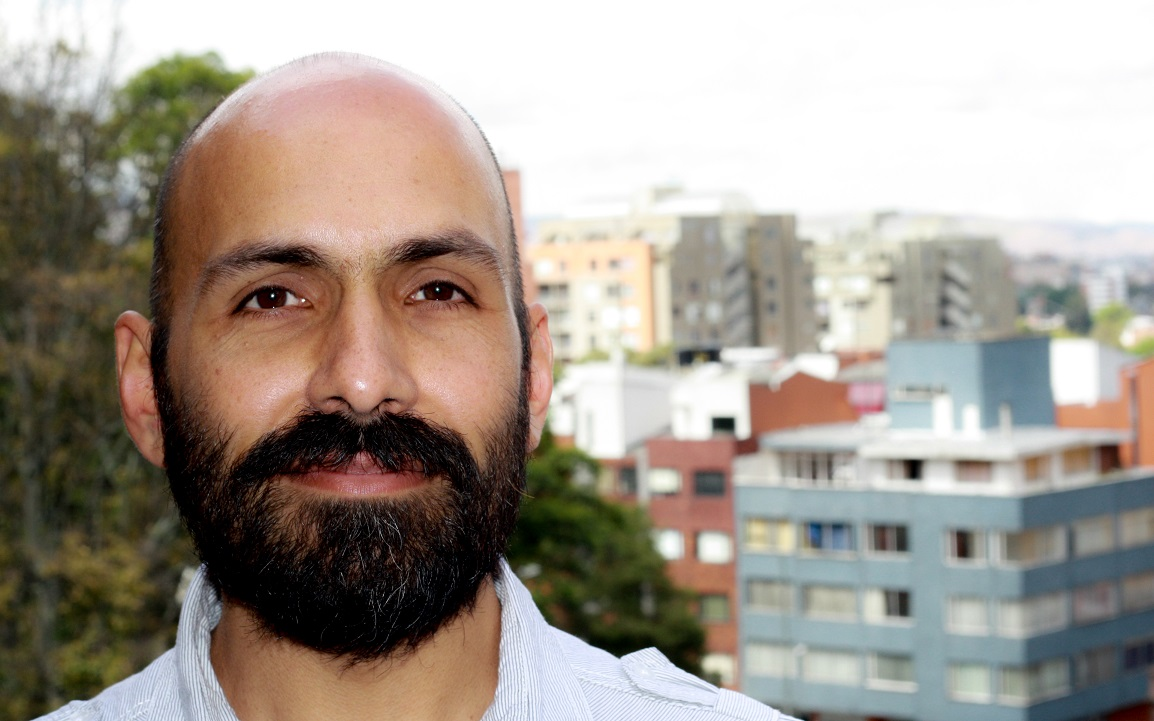Plazas shows that the bigger Connes-Marcolli $GL_2$-system also acts on the Big Picture. An intriguing quote:

“Our interest in the $GL_2$-system comes from the fact that its thermodynamic properties encode the arithmetic theory of modular functions to an extend which makes it possible for us to capture aspects of moonshine theory.”

Looks like the right kind of paper to take along when I disappear next week for some time in the French mountains…

One of the better opening quotes of a paper:

“Even quite ungainly objects, like chairs and tables, will become almost spherical if you wrap them in enough newspaper.”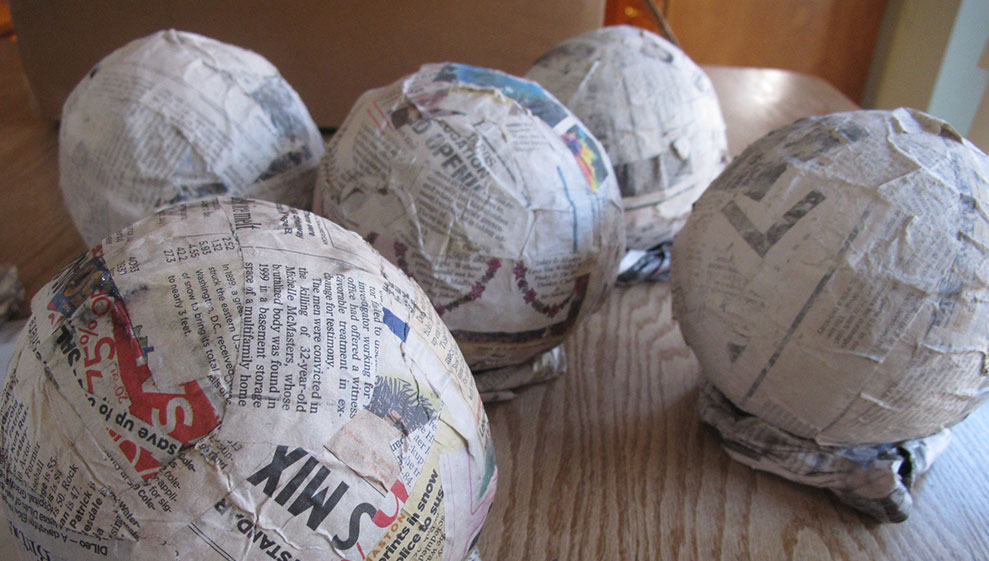The paper in question is The orbifold notation for surface groups by John Conway.

Here’s Conway talking leisurely about Thurston’s idea to capture the acting group via the topology of the orbifold space and his own notation for such orbifolds.

Here’s another version of the paper, with illustrations: The orbifold notation for two-dimensional groups, by Conway and Daniel H. Huson.

A very accessible account are these lecture notes:

A field guide to the orbifolds, notes from class on “Geometry and the Imagination” in Minneapolis, with John Conway, Peter Doyle, Jane Gilman and Bill Thurston, on June 17–28, 1991.

And, here are notes by Thurston on The Geometry and Topology of Three-Manifolds, including stuff about orbifolds.

I came across these papers struggling my way through On the discrete groups of moonshine by Conway, McKay and Sebbar.

On the genus $0$ property of moonshine groups they have this to say:

“As for groups of the form $(n|h)+e,f,\dots$, the genus can be determined from the fundamental regions using the Riemann-Hurwitz formula. Since most of the groups are not subgroups of the modular group, the calculations of the genus, which cannot be produced here because of their length, are carried out by finding the elliptic fixed points and the cone points in the orbifolds attached to the fundamental regions. The Euler characteristic of the orbifold determines the genus of the group. See [paper] for more details on orbifold techniques.”

Next week, the brand new séminaire « Lectures grothendieckiennes » will kick off on Tuesday October 24th at 18hr (h/t Isar Stubbe).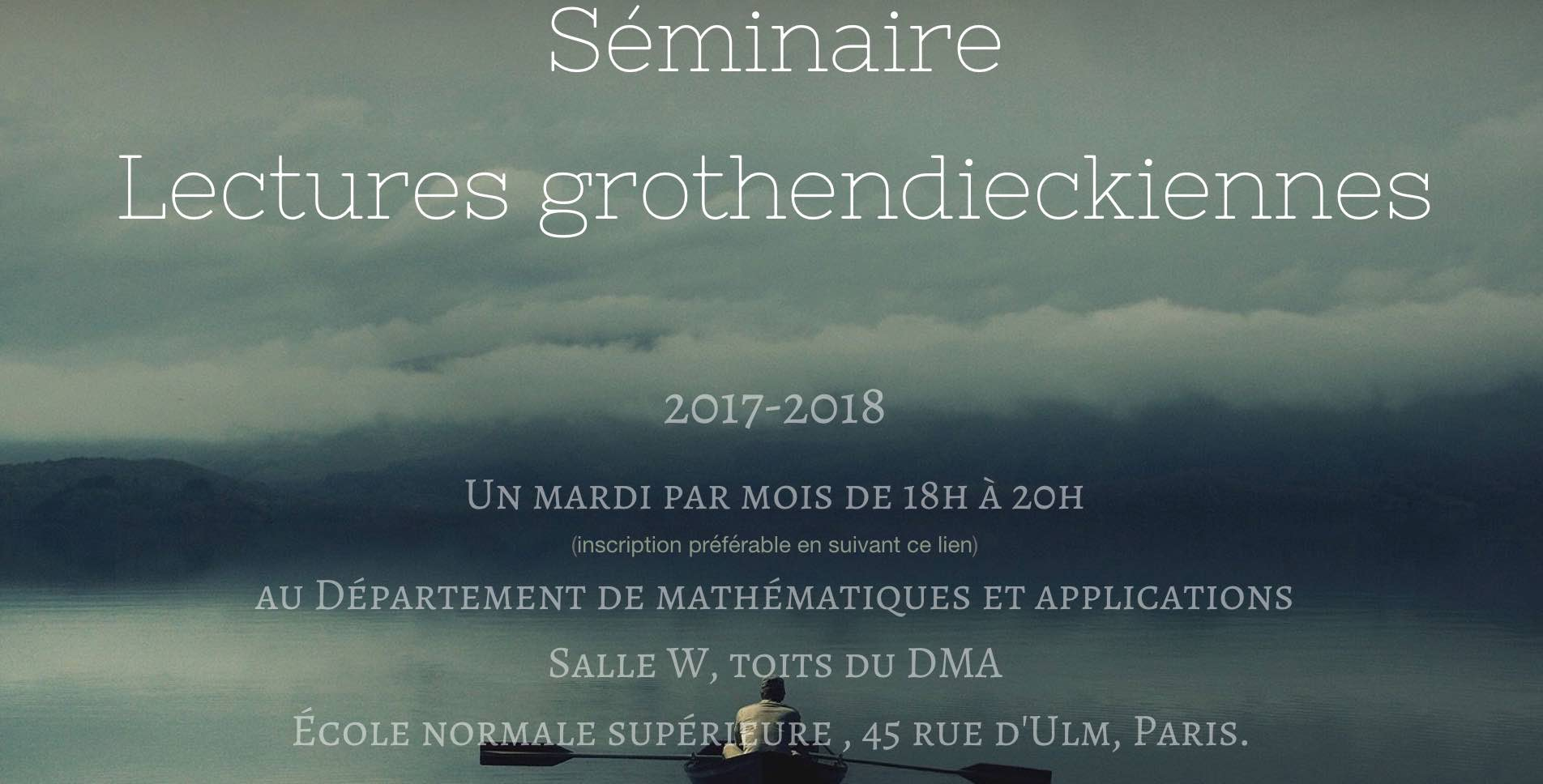There will be one talk a month, on a tuesday evening from 18hr-20hr. Among the lecturers are the ‘usual suspects’:

Pierre Cartier (October 24th) will discuss the state of functional analysis before Grothendieck entered the scene in 1948 and effectively ‘killed the subject’ (said Dieudonné).

Alain Connes (November 7th) will talk on the origins of Grothendieck’s introduction of toposes.

In fact, toposes will likely be a recurrent topic of the seminar.

Laurant Laforgue‘s title will be ‘La notion de vérité selon Grothendieck'(January 9th) and on March 6th there will be a lecture by Olivia Caramello.

Also, Colin McLarty will speak about them on May 3rd: “Nonetheless one should learn the language of topos: Grothendieck on building houses”.

The closing lecture will be delivered by Georges Maltsiniotis on June 5th 2018.

Further Grothendieck news, there’s the exhibition of a sculpture by Nina Douglas, the wife of Michael Douglas, at the Simons Center for Geometry and Physics (h/t Jason Starr).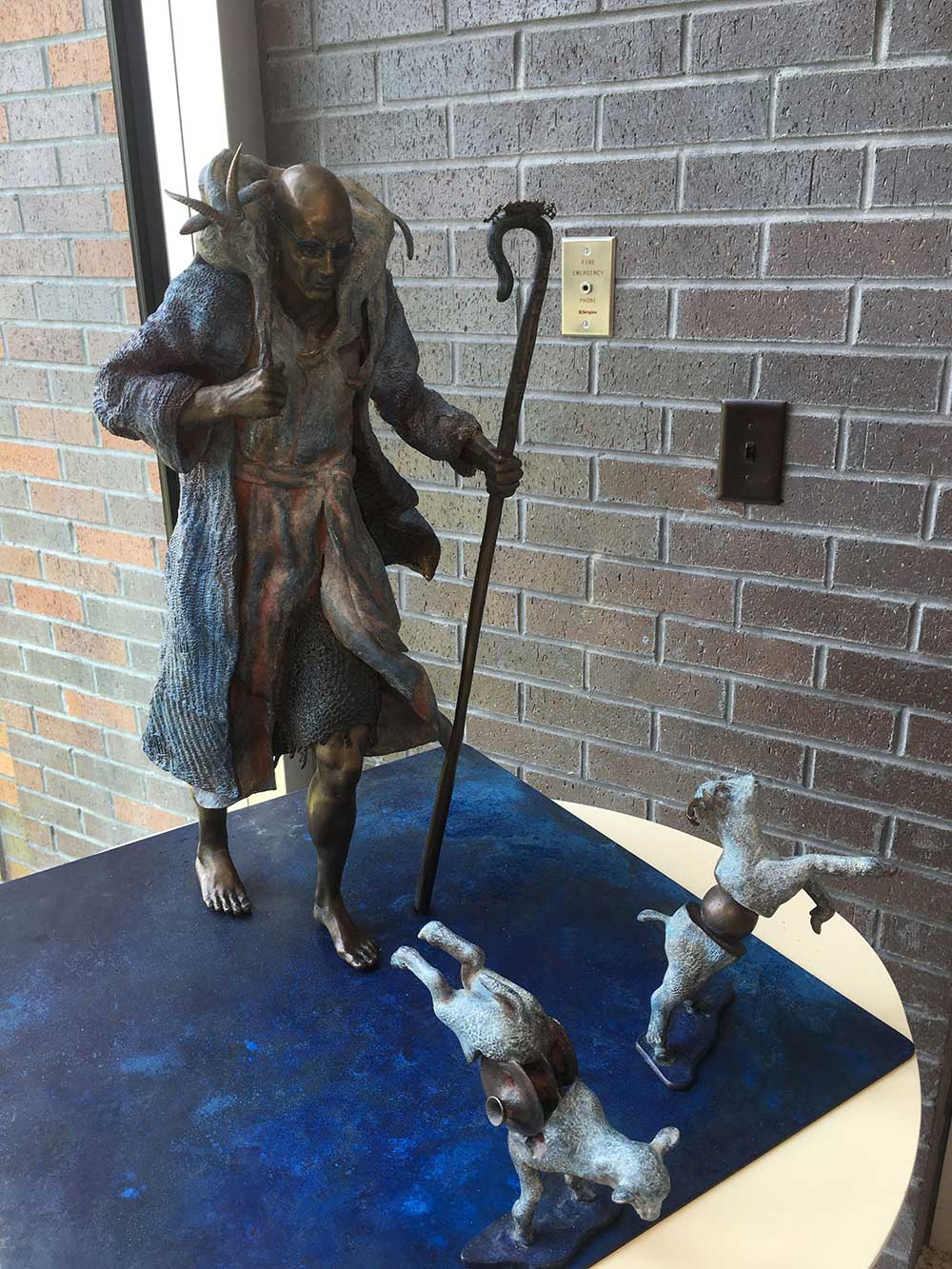It depicts Grothendieck as shepherd. The lambs in front of him have Riemann surfaces inserted into them and on the staff is Grothendieck’s ‘Hexenkuche’ (his proof of the Riemann-Roch theorem).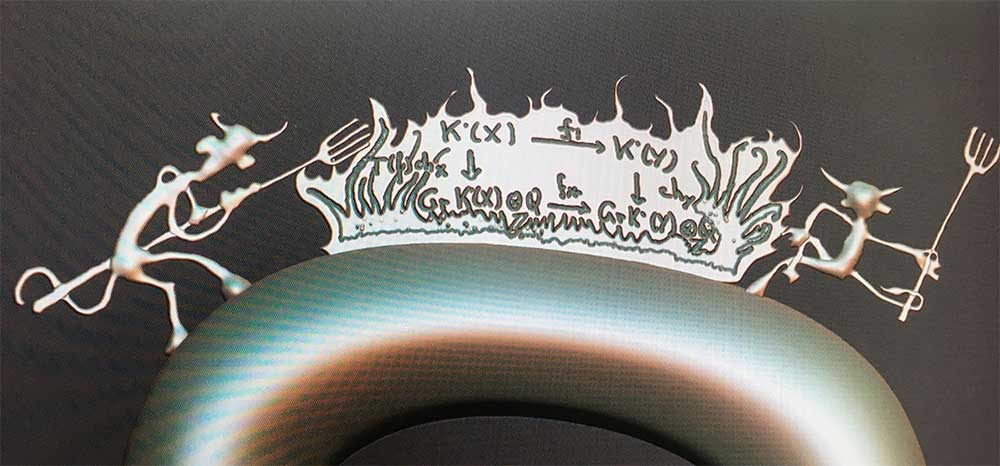In several of his talks on #IUTeich, Mochizuki argues that usual scheme theory over $\mathbb{Z}$ is not suited to tackle problems such as the ABC-conjecture.

The idea appears to be that ABC involves both the additive and multiplicative nature of integers, making rings into ‘2-dimensional objects’ (and clearly we use both ‘dimensions’ in the theory of schemes).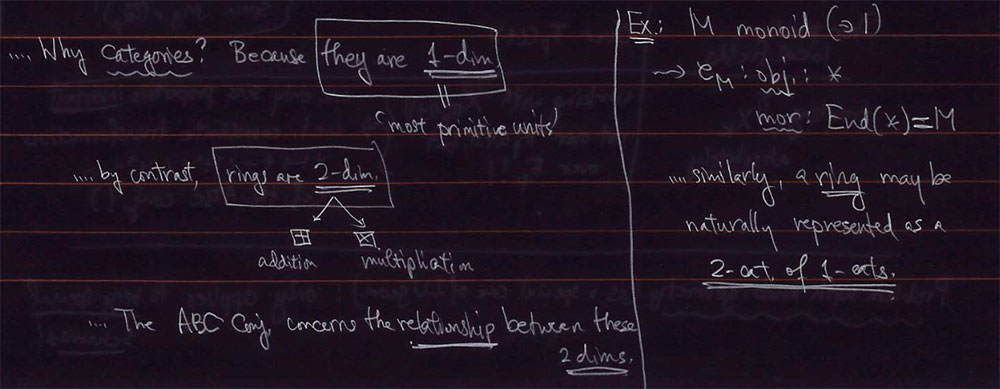So, perhaps we should try to ‘dismantle’ scheme theory, and replace it with something like geometry over the field with one element $\mathbb{F}_1$.

The usual $\mathbb{F}_1$ mantra being: ‘forget all about the additive structure and only retain the multiplicative monoid’.

So perhaps there is yet another geometry out there, forgetting about the multiplicative structure, and retaining just the addition…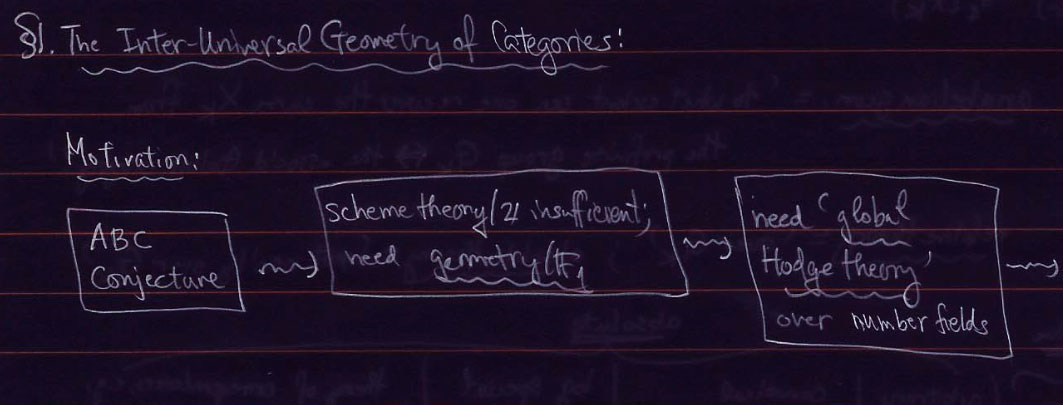In the forgetting can’t be that hard, can it?-post we have seen that the forgetful functor

$F_{+,\times}~:~\mathbf{rings} \rightarrow \mathbf{sets}$

(that is, forgetting both multiplicative and additive information of the ring) is representable by the polynomial ring $\mathbb{Z}[x]$.

So, what about our ‘dismantling functors’ in which we selectively forget just one of these structures:

$F_+~:~\mathbf{rings} \rightarrow \mathbf{monoids} \quad \text{and} \quad F_{\times}~:~\mathbf{rings} \rightarrow \mathbf{abelian~groups}$

Are these functors representable too?

Clearly, ring maps from $\mathbb{Z}[x]$ to our ring $R$ give us again the elements of $R$. But now, we want to encode the way two of these elements add (or multiply).

This can be done by adding extra structure to the ring $\mathbb{Z}[x]$, namely a comultiplication $\Delta$ and a counit $\epsilon$

$\Delta~:~\mathbb{Z}[x] \rightarrow \mathbb{Z}[x] \otimes \mathbb{Z}[x] \quad \text{and} \quad \epsilon~:~\mathbb{Z}[x] \rightarrow \mathbb{Z}$

The idea of the comultiplication being that if we have two elements $r,s \in R$ with corresponding ring maps $f_r~:~\mathbb{Z}[x] \rightarrow R \quad x \mapsto r$ and $f_s~:~\mathbb{Z}[x] \rightarrow R \quad x \mapsto s$, composing their tensorproduct with the comultiplication

$f_v~:~\mathbb{Z}[x] \rightarrow^{\Delta} \mathbb{Z}[x] \otimes \mathbb{Z}[x] \rightarrow^{f_r \otimes f_s} R$

determines another element $v \in R$ which we can take either the product $v=r.s$ or sum $v=r+s$, depending on the comultiplication map $\Delta$.

The role of the counit is merely sending $x$ to the identity element of the operation.

Thus, if we want to represent the functor forgetting the addition, and retaining the multiplication we have to put on $\mathbb{Z}[x]$ the structure of a biring

$\Delta(x) = x \otimes x \quad \text{and} \quad \epsilon(x) = 1$

(making $x$ into a ‘group-like’ element for Hopf-ists).

The functor $F_{\times}$ forgetting the multiplication but retaining the addition is represented by the Hopf-ring $\mathbb{Z}[x]$, this time with

$\Delta(x) = x \otimes 1 + 1 \otimes x \quad \text{and} \quad \epsilon(x) = 0$

(that is, this time $x$ becomes a ‘primitive’ element).

Perhaps this adds another feather of weight to the proposal in which one defines algebras over the field with one element $\mathbb{F}_1$ to be birings over $\mathbb{Z}$, with the co-ring structure playing the role of descent data from $\mathbb{Z}$ to $\mathbb{F}_1$.

As, for example, in my note The coordinate biring of $\mathbf{Spec}(\mathbb{Z})/\mathbb{F}_1$.

Soon, we will be teaching computational geometry courses to football commentators.

If a player is going to be substituted we’ll hear sentences like: “no surprise he’s being replaced, his Voronoi cell has been shrinking since the beginning of the second half!”

David Sumpter, the author of Soccermatics: Mathematical Adventures in the Beautiful Game, wrote a nice article over at Medium The geometry of attacking football.

As an example, he took an attack of Barcelona against Panathinaikos.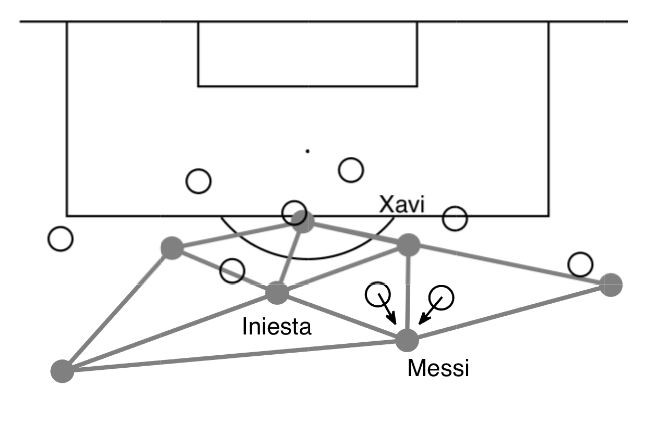and explained the passing possibilities in terms of the Delaunay triangulation between the Barca-players (the corresponding Voronoi cell decomposition is in the header picture).

He concludes: “It is not only their skill on the ball, but also their geometrically accurate positioning that allows them to make the pass.”

Jaime Sampaoi produced a short video of changing Voronoi cells from kick-off by the blue team, with the red team putting pressure until a faulty pass is given, leading to a red-attack and a goal. All in 29 seconds.

I’d love to turn this feature on when watching an actual game.

Oh, and please different cell-colours for the two teams.

And, a remote control to highlight the Voronoi cell of a particular player.